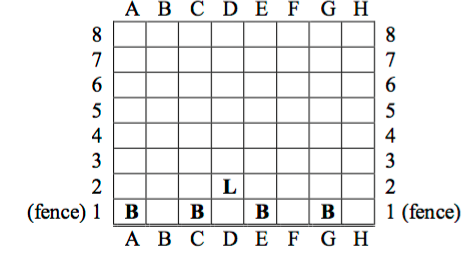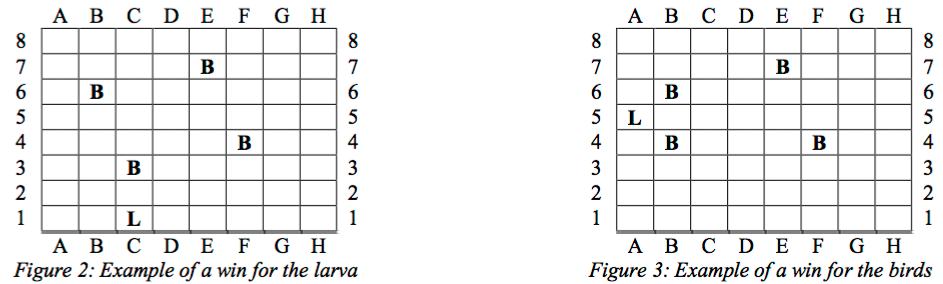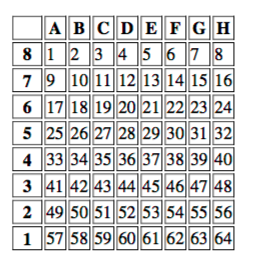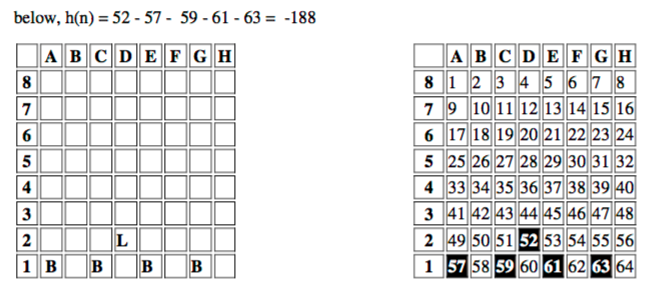# Better Heuristic function for a game (AI Minimax)

1 view

There is a game that I've programmed in java. The game is simple (refer to the figure below). There are 4 birds and 1 larva. It is a 2 player game (AI vs Human).• The larva can move diagonally forward AND diagonally backward

• Birds can ONLY move diagonally forward

• Larva wins if it can get to line 1 (fence)

• Larva also wins if birds have no moves left

• Birds CANNOT "eat" the larva.

• Birds win if Larva has NO move left (cannot move at all)When the game starts, Larva begins, then ONE bird can move (anyone), then Larva, etc...

I have implemented a MiniMax (Alpha Beta Pruning) and I'm using the following evaluate() function (heuristic function).

Let us give the following numbers to each square on the board.Therefore, our evaluation function will be

h(n) = value of the position of larva - the value of the position of bird 1 - the value of the position of bird 2 - the value of the position of bird 3 - the value of the position of bird 4

the Larva will try to MAXIMIZE the heuristic value whereas the Birds will try to MINIMIZe it

Example:However, this is a simple and naive heuristic. It does not act smartly. I am a beginner in AI and I would like to know what can I do to IMPROVE this heuristic function?

What would be a good/informed heuristic?

by (81.7k points)

In your current heuristic, the values of square A1 is 8 less than the value of square A8. This makes the Birds inclined to move towards the left side of the game board, as a move to the left will always be higher than a move to the right. This is not accurate. All squares on row 1 should have the same value. Thus assign all squares in row 1 a 1, in row 2 a 2, etc. This way the birds and larva won't be inclined to move to the left, and instead can focus on making a good move.

You could take into account the fact that birds will have a positional advantage over the larva when the larva is on the sides of the board, so if Larva is MAX then you can change the side tile values of the board to be smaller.

Minimax is a decision rule used in Artificial Intelligence so if you wish to learn more about Minimax Decision Rule then visit this Artificial Intelligence Course.